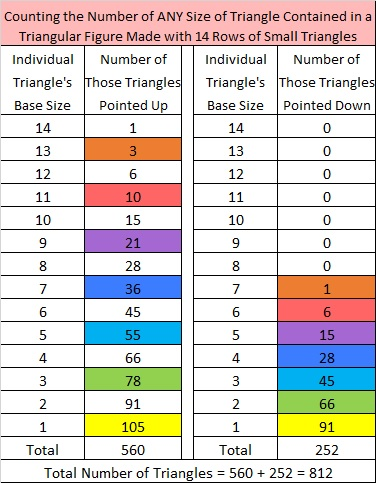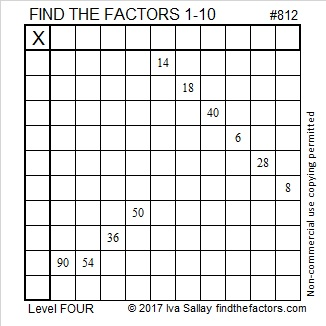# 812 How Many Triangles in All?

Here’s a puzzle for you. How many total triangles are there in the figure below?Okay, if you guessed 812 because this is my 812th post, you would be right. But what if you were asked that question in some real world situation where accessing the internet to get the answer isn’t permitted. How would you know the answer then?

True, you might have memorized the formula I mentioned in 658-How Many Triangles Point Up? How Many Triangles Point Down? How Many Triangles in All?:

• The total number of triangles = ⌊n(n+2)(2n+1)/8⌋ where the brackets mean round decimals DOWN to the closest integer.
• Here n = 14, so the number of triangles is 14×16×29/8 = 812. Rounding down wasn’t necessary since the product of two consecutive even numbers is always divisible by 8.

Still, you probably wouldn’t remember that formula unless you had seen it VERY recently or you have a photographic memory.

You could actually COUNT all the triangles. In the post about 658 total triangles, I noted that the 13 rows of small triangles formed a total of 169 of the smallest triangles, but I suggested that it would be easier to ignore that nice square number and instead count the number of triangles pointing UP separately from the number pointing DOWN. You will add up a lot of triangular numbers as you sum up the number of them pointing up and again as you sum up the number pointing down.

Making a chart of the number of triangles pointing UP would be easy. It’s just a list of triangular numbers in order. However, the chart for the ones pointing down might be confusing because you don’t use all of the triangular numbers, and the ones you use will be different for an even number of rows than for an odd number of rows. For example,

• the pointing DOWN portion of the chart for 14 rows of triangles below uses these seven triangular numbers: 1, 6, 15, 28, 45, 66, and 91,
• while the pointing DOWN chart for 13 rows of triangles uses six different triangular numbers: 3, 10, 21, 36, 55, and 78.

Here is a chart listing the number of triangles of any size that are contained in a triangular figure made with 14 rows of small triangles. Interesting note: Because 14 + 2 = 16, a multiple of 8, the total number of triangles in this case will be divisible by 14, the number of line segments on each side.Making such a chart works. However, remembering  which triangular numbers to use and how many you should use, especially when counting the odd number of rows of triangles pointing DOWN, might be difficult.

Today I was thinking about triangular numbers and their relationship to square numbers:

• The sum of two consecutive triangular numbers always makes a square number. On the chart above, I’ve paired up certain consecutive triangular numbers by coloring them the same color. The sizes of the triangles being paired together by like colors are not the same size (with one exception), but their sums are nevertheless square numbers every time.
• Thus, we also can come up with a single list of numbers to add to arrive at the total number of triangles as illustrated below. This has made me changed my mind about using those square numbers to help count! Notice how every other number on each list below, including the last number to be added, is a square number.Of the methods discussed in this post, this one that includes square numbers might be the easiest one to remember. Here are the steps I used to find the total number of triangles:

• Count the rows and determine if that number is even or odd.
• Write the triangular numbers in order until the amount of numbers written equals the number of rows.
• If the number of rows is even, replace the 2nd, 4th, 6th, etc. entries with corresponding square numbers: 4, 16, 36, etc.
• If the number of rows is odd, replace the 1st, 3rd, 5th, etc entries with corresponding square numbers: 1, 9, 25, etc.
• Add all the numbers remaining in the list. The sum will be the total number of triangles for that many rows of small triangles.

Okay, that puzzle was rather difficult and took a while to explain. You may find this Level 4 puzzle easier:Print the puzzles or type the solution on this excel file: 10-factors 807-814

Here’s a little more about the number 812:

• 812 is a composite number.
• Prime factorization: 812 = 2 x 2 x 7 x 29, which can be written 812 = 2² x 7 x 29
• The exponents in the prime factorization are 2, 1, and 1. Adding one to each and multiplying we get (2 + 1)(1 + 1)(1 + 1) = 3 x 2 x 2 = 12. Therefore 812 has exactly 12 factors.
• Factors of 812: 1, 2, 4, 7, 14, 28, 29, 58, 116, 203, 406, 812
• Factor pairs: 812 = 1 x 812, 2 x 406, 4 x 203, 7 x 116, 14 x 58, or 28 x 29
• Taking the factor pair with the largest square number factor, we get √812 = (√4)(√203) = 2√203 ≈ 28.4956 (That was three multiples of 7)812 = 28 × 29, which means it is the sum of the first 28 even numbers.

• Thus, 2 + 4 + 6 + 8 + . . .  + 52 + 54 + 56 = 812

It also means that we are halfway between 28² and 29², or halfway between 784 and 841. The average of those two numbers is 812.5.

AND it means that 28² + 29² – 1 = 2(812) = 2(28 × 29)

Since 29 is one of its factors, 812 is also the hypotenuse of a Pythagorean triple:

• 560-588-812 which is 28 times 20-21-29.## 2 thoughts on “812 How Many Triangles in All?”

1.wbhs62

Well, well, well. Or, if you prefer, Wow, wow, wow!

•ivasallay

Thank you! I like both exclamations.

This site uses Akismet to reduce spam. Learn how your comment data is processed.